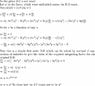# Easier way of finding Integrating Factor for Exact Differential Equation?

• pr0me7heu2

#### pr0me7heu2

Easier way of finding Integrating Factor for Exact Differential Equation??

## Homework Statement

,find an integrating factor and then solve the following:

[4(x3/y2)+(3/y)]dx + [3(x/y2)+4y]dy = 0

## Homework Equations

u(y)=y2 is a valid integrating factor that yields a solution x4+3xy+y4=c

,no clue how though!

## The Attempt at a Solution

I understand exact differential equations to really be a somewhat perverted form of a gradient of a multivariable surface defined by f=f(x,y)... and that solving an exact differential equations is comparable to finding the potential function using the fundamental theorem of line integrals.

,here with this equation I tried the usual methods

I first realized that the equation is NOT exact (My does not equal Nx), or in other words the two parts of the differential equation could not have possibly come from the same function f=f(x,y) in their current forms because fxy=fyx for any f(x,y).

So, I multiplied by an integrating factor u(x,y) such that the differential equation would be exact or such that (uM)y=(uN)x

,so far in my math education I've pretty much disregarded the idea of finding an integrating factor that is not solely a function of either y or x - it simply would be too hard.

,so in assuming u to be a function of either solely x or y I had hoped to find a linear and separable DE through the following:

du/dx=[u(My-Nx]/N or

du/dy=[u(Nx-My]/M

,unfortunately both of these yield seemingly impossibly, or at least algebraically disgusting results...

Any ideas on an easier or other method for solving for this/other integrating factors?

,and on a slightly related note, any good referenced here in the forums for writing better math symbols?

Um, doesn't multiplying the entire expression by y^2 gives you a form which is exact? Why do you say you have no clue when you know the correct method? Once you have reduced the DE to an exact one, then you can simply find the potential function, which you appear to have already done. So what's the problem here?

...because the method of haphazrdly looking at the equation and thinking, "what will make this exact?" isn't exactly a method that will work all the time. And the method presented in the book and class yields factors that would simply suck trying to integrate.

I was just curious if there were any other strategies that anyone knew of.

,so as a revision to my original post... I understand how and why y2 is a factor - I just don't exactly see how it was reached.

Last edited:

I have a question on this problem...

I can see why y2 is an integrating factor, but I don't know how this answer can be derived. Do you use:

$$\frac{N_{x}(x,y) - M_{y}(x,y)}{M(x,y)}$$
Where M (x,y) = 4(x3/y2)+(3/y)]dx, and N = 3(x/y2)+4y dy

When I try and solve this, I get a very complex integrating factor.

See the attachment for how $$y^{2}$$ comes out as the integrating factor for the given D.E

#### Attachments

•result1.jpg
37.5 KB · Views: 750